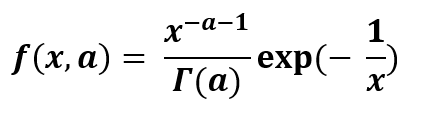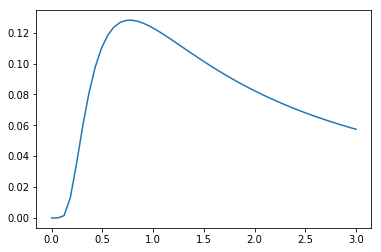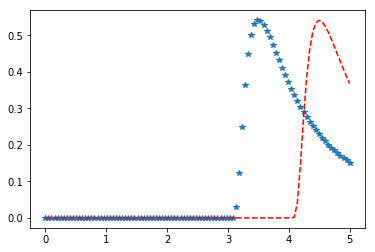# Inverse Gamma Distribution in Python

Inverse Gamma distribution is a continuous probability distribution with two parameters on the positive real line. It is the reciprocate distribution of a variable distributed according to the gamma distribution. It is very useful in Bayesian statistics as the marginal distribution for the unknown variance of a normal distribution. It is used for considering the alternate parameter for the normal distribution in terms of the precision which is actually the reciprocal of the variance.``` scipy.stats.invgamma() : ```

` ` It is an inverted gamma continuous random variable. It is an instance of the `rv_continuous` class. It inherits from the collection of generic methods and combines them with the complete specification of distribution.

Code #1 : Creating inverted gamma continuous random variable

 `from` `scipy.stats ``import` `invgamma   ` `   `  `numargs ``=` `invgamma.numargs ` `[a] ``=` `[``0.3``] ``*` `numargs ` `rv ``=` `invgamma (a) ` `   `  `print` `(``"RV : \n"``, rv)  `

Output :

RV :
scipy.stats._distn_infrastructure.rv_frozen object at 0x00000230B0B28748

Code #2 : Inverse Gamma continuous variates and probability distribution

 `import` `numpy as np ` `quantile ``=` `np.arange (``0.01``, ``1``, ``0.1``) ` `    `  `# Random Variates ` `R ``=` `invgamma.rvs(a, scale ``=` `2``,  size ``=` `10``) ` `print` `(``"Random Variates : \n"``, R) ` `   `  `# PDF ` `R ``=` `invgamma .pdf(a, quantile, loc ``=` `0``, scale ``=` `1``) ` `print` `(``"\nProbability Distribution : \n"``, R) `

Output :

```Random Variates :
[4.18816252e+00 2.02807957e+03 8.37914946e+01 1.94368997e+00
3.78345091e+00 1.00496176e+06 3.42396458e+03 3.45520522e+00
2.81037118e+00 1.72359706e+03]

Probability Distribution :
[0.0012104  0.0157619  0.03512042 0.05975504 0.09007126 0.12639944
0.16898506 0.21798098 0.27344182 0.33532072]```

Code #3 : Graphical Representation.

 `import` `numpy as np ` `import` `matplotlib.pyplot as plt ` `   `  `distribution ``=` `np.linspace(``0``, np.minimum(rv.dist.b, ``3``)) ` `print``(``"Distribution : \n"``, distribution) ` `   `  `plot ``=` `plt.plot(distribution, rv.pdf(distribution)) `

Output :

```Distribution :
[0.         0.06122449 0.12244898 0.18367347 0.24489796 0.30612245
0.36734694 0.42857143 0.48979592 0.55102041 0.6122449  0.67346939
0.73469388 0.79591837 0.85714286 0.91836735 0.97959184 1.04081633
1.10204082 1.16326531 1.2244898  1.28571429 1.34693878 1.40816327
1.46938776 1.53061224 1.59183673 1.65306122 1.71428571 1.7755102
1.83673469 1.89795918 1.95918367 2.02040816 2.08163265 2.14285714
2.20408163 2.26530612 2.32653061 2.3877551  2.44897959 2.51020408
2.57142857 2.63265306 2.69387755 2.75510204 2.81632653 2.87755102
2.93877551 3.        ]```Code #4 : Varying Positional Arguments

 `import` `matplotlib.pyplot as plt ` `import` `numpy as np ` `   `  `x ``=` `np.linspace(``0``, ``5``, ``100``) ` `   `  `# Varying positional arguments ` `y1 ``=` `invgamma .pdf(x, ``1``, ``3``) ` `y2 ``=` `invgamma .pdf(x, ``1``, ``4``) ` `plt.plot(x, y1, ``"*"``, x, y2, ``"r--"``) `

Output :Attention geek! Strengthen your foundations with the Python Programming Foundation Course and learn the basics.

To begin with, your interview preparations Enhance your Data Structures concepts with the Python DS Course.

My Personal Notes arrow_drop_upCheck out this Author's contributed articles.

If you like GeeksforGeeks and would like to contribute, you can also write an article using contribute.geeksforgeeks.org or mail your article to contribute@geeksforgeeks.org. See your article appearing on the GeeksforGeeks main page and help other Geeks.

Please Improve this article if you find anything incorrect by clicking on the "Improve Article" button below.

Article Tags :
Practice Tags :

Be the First to upvote.

Please write to us at contribute@geeksforgeeks.org to report any issue with the above content.